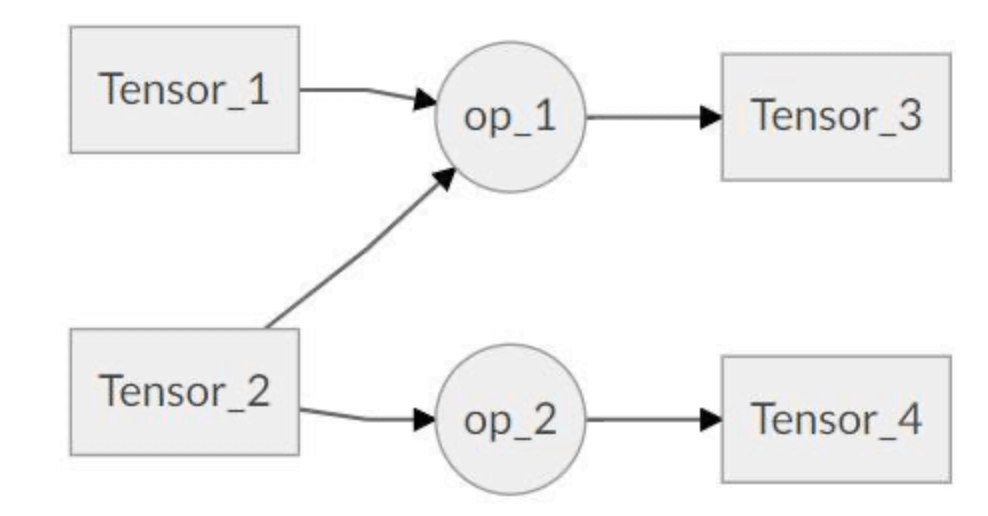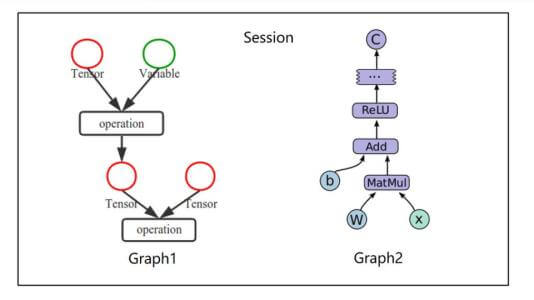# 【Tensorflow基础】第二课：Tensorflow基本概念

## Graph，Session，Tensor，Operation，Feed，Fetch

Posted by x-jeff on February 20, 2020

# 1.Tensorflow基本概念

## 1.1.Graph

Graph（图）：表示计算任务，用于搭建神经网络的计算任务。

## 1.2.Session

Session（会话）：在Session中执行Graph。

## 1.3.Tensor

Tensor（张量）：张量就是一种拥有不同维度的数据结构。Tensor是Tensorflow中的基本数据结构：

• 0阶张量（标量）：一个数。
• 1阶张量（向量）：一维数组。
• 2阶张量（矩阵）：二维数组。
• ……（以此类推）

⚠️Tensorflow中的一切数据都属于Tensor类型。

⚠️任何Tensor数据在运算之前都是得不到具体数值的。

1. 可更新的参数：包括权重（weights），偏置项（bias）。这些参数将在训练过程中不断更新。
• 对应数据类型：tf.Variable
2. 独立于模型存在的数据：数据集中的数据需要“喂给”网络，包括输入数据、输出端的groundtruth。
• 对应数据类型：tf.placeholder
3. 常量。
• 对应数据类型：tf.constant

## 1.4.Operation

Operation（操作）：图中的节点称之为op(operation)。但凡是op，都需要通过Session运行之后，才能得到结果。

⚠️Graph是由一系列op构成的。

• Tensor对象的op属性指向生成该Tensor的Operation对象。
• Tensor对象的consumers()函数获取使用该Tensor对象作为输入的Operation对象。
• Operation对象的inputs属性指向该计算节点的输入Tensor对象。
• Operation对象的outputs属性指向该计算节点的输出Tensor对象。## 1.6.例子1
2
3
import tensorflow as tf
tensor1 = tf.constant([[3,4]])
tensor2 = tf.constant([,])


tensor1是一个$1\times 2$维的向量，tensor2是一个$2\times 1$维的向量。现在新建一个op，计算两个tensor的点积：

1
2
tensor3 = tf.matmul(tensor1,tensor2)
print(tensor3)


❗️tf.matmul()可用于计算点积。

1
2
3
4
5
6
7
#定义一个新的Session
sess = tf.Session()
#开始计算Graph
result = sess.run(tensor3)
print(result)
#关闭Session
sess.close()


1
2
3
with tf.Session() as sess:
result = sess.run(tensor3)
print(result)


# 2.Variable

1
2
3
4
5
6
7
8
9
10
11
12
13
14
import tensorflow as tf
v1 = tf.Variable([1,2])
c1 = tf.constant([3,4])
sub = tf.subtract(v1,c1) #定义一个减法op

init = tf.global_variables_initializer() #初始化全局变量

with tf.Session() as sess:
sess.run(init)
sub_result = sess.run(sub)
print(sub_result) #[-2,-2]


‼️Variable必须初始化：

• init = tf.global_variables_initializer()可以初始化全局所有变量。
• sess.run(v1.initializer)只初始化变量v1

1
2
3
4
5
6
7
8
9
v2 = tf.Variable(0,name="counter")
update_v2 = tf.assign(v2,new_v2) #用于赋值操作
init = tf.global_variables_initializer() #初始化全局变量
with tf.Session() as sess:
sess.run(init)
for _ in range(5):
sess.run(update_v2)
print(sess.run(v2))


1
2
3
4
5
1
2
3
4
5


# 3.Feed、Fetch

## 3.1.Fetch

Fetch字面意思是“取来”，即得到运行结果。在sess.run()中，即可fetch一个op的值，也可同时fetch多个op的值。

1
2
3
4
5
6
7
8
c1=tf.constant(1)
c2=tf.constant(2)
c3=tf.constant(3)
with tf.Session() as sess:
print(result1) #[5,5]


⚠️sess.run([mul1,add1])是以列表list的形式，因此不要忘了[]

## 3.2.Feed

Feed字面意思是“喂养”，即喂入数据。

1
2
3
4
5
6
p1=tf.placeholder(tf.float32)
p2=tf.placeholder(tf.float32)
mul2=tf.multiply(p1,p2)
with tf.Session() as sess:
result2=sess.run(mul2,feed_dict={p1:[5.],p2:[.3]})
print(result2)


⚠️Feed操作是通过python字典的形式传入数据的。

• sess.run(mul2,feed_dict={p1:[5.],p2:[.3]})输出为一个numpy数组ndarray：[1.5]。
• sess.run(mul2,feed_dict={p1:5.,p2:.3})输出为一个数值float：1.5。

# 5.参考资料